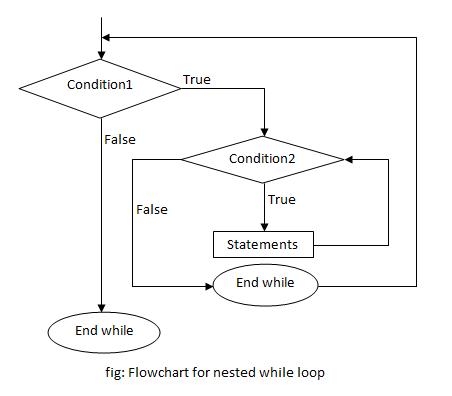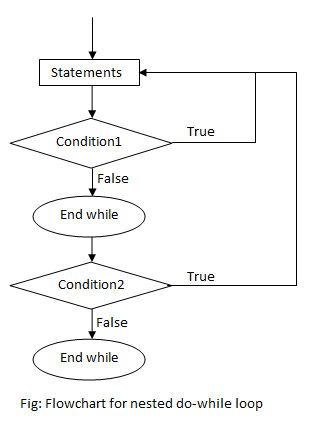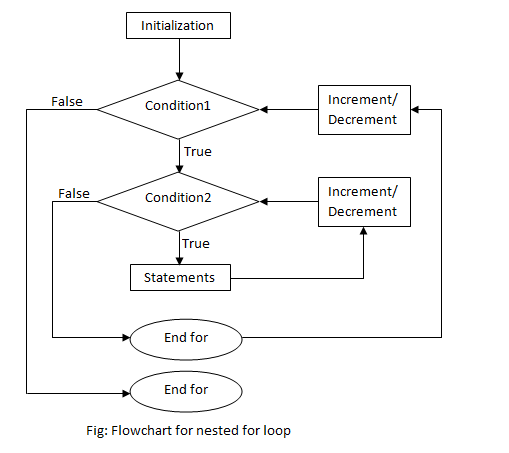# Nested loops in C++ Programming

A loop inside another loop is called a nested loop. The number of loops depend on the complexity of a problem. Suppose, a loop, outer loop, running n number of times consists of another loop inside it, inner loop, running m number of times. Then, for each execution of the outer loop from 1…n, the inner loop runs maximum of m times.

## Types of nested loops

There can be many types of nested loops in C++ but the mostly used nested loops are

Note: There can also be very variation of nested loops where a while loop can be inside a for loop, a for loop can be inside a do-while loop and many more.

## Nested while loop

A while loop inside another while loop is called nested while loop.

### Syntax of Nested while loop

```while (condition1)
{
statement(s);
while (condition2)
{
statement(s);
... ... ...
}
... ... ...
}```

### Flowchart for Nested while loop### Example of Nested while loop

C++ program to print the number pattern.

```1
1 2
1 2 3
1 2 3 4
1 2 3 4 5```
```#include <iostream>
#include <conio.h>
using namespace std;

int main()
{
int i=1,j;
while (i <= 5)
{
j=1;
while (j <= i )
{
cout <<j;
j++;
}
cout << endl;
i++;
}
getch();
return 0;
}```

In this program, nested while loop is used to print the pattern. The outermost loop runs 5 times and for every loop, the innermost loop runs i times which is 1 at first, meaning only “1” is printed, then on the next loop it’s 2 numbers printing “1 2” and so on till 5 iterations of the loop executes, printing “1 2 3 4 5”. This way, the given number pattern is printed.

## Nested do-while loop

A do-while loop inside another do-while loop is called nested do-while loop.

### Syntax of Nested do-while loop

```do
{
statement(s);
do
{
statement(s);
... ... ...
}while (condition2);
... ... ...
}while (condition1);```

### Flowchart of Nested do-while loop### Example of do-while loop

C++ program to print the given star pattern.

```*
**
***
****
*****```
```#include <iostream>
#include <conio.h>
using namespace std;

int main()
{
int i=1,j;
do
{
j=1;
do
{
cout << "*";
j++;
}while(j <= i);
i++;
cout << endl;
}while(i <= 5);
getch();
return 0;
}```

In this program, nested do-while loop is used to print the star pattern. The outermost loop runs 5 times and for every loop, the innermost loop runs i times which is 1 at first, meaning only one “*” is printed, then on the next loop it’s 2 printing two stars and so on till 5 iterations of the loop executes, printing five stars. This way, the given star pattern is printed.

## Nested for loop

A for loop inside another for loop is called nested for loop.

### Syntax of Nested for loop

```for (initialization; condition; increment/decrement)
{
statement(s);
for (initialization; condition; increment/decrement)
{
statement(s);
... ... ...
}
... ... ...
}```

### Flowchart of Nested for loop### Example of Nested for loop

C++ program to find the sum of 2 matrices.

```#include <iostream>
#include <conio.h>
using namespace std;

int main()
{
int a, b, s;
int i,j,row, column;
cout <<"Enter size of row:";
cin >> row;
cout <<"Enter size of column:";
cin >> column;
cout <<"Enter elements of matrix A" << endl;
for(i=0;i<row;i++)
{
for(j=0;j<column;j++)
{
cin >> a[i][j];
}
}
cout <<"Enter elements of matrix B" << endl;
for(i=0;i<row;i++)
{
for(j=0;j<column;j++)
{
cin >> b[i][j];
}
}
cout << "Sum of A and B" << endl;
for(i=0;i<row;i++)
{
for(j=0;j<column;j++)
{
cout << a[i][j] + b[i][j] << "   ";
}
cout << endl;
}
getch();
return 0;
}```

Nested for loop is used to calculate the sum of two 2-dimensional matrices. The program consists of three for nested loops where the outer loop runs equal to size of row and inner loop runs equal to size of column. The first and second are used for entering the values of elements for Matrix A and B, while the third is used for displaying the sum of the elements of the two matrices. Matrix A and B are stored in 2-dimensional arrays a and b respectively. In the final nested loop, each element of a and b is traversed and the sum is printed.

Output

```Enter size of row:2
Enter size of column:3
Enter elements of matrix A
2 7 0
3 -1 7
Enter elements of matrix B
4 9 2
0 1 -8
Sum of A and B
6   16   2
3   0   -1```